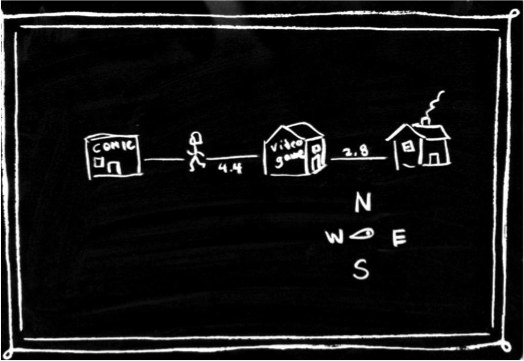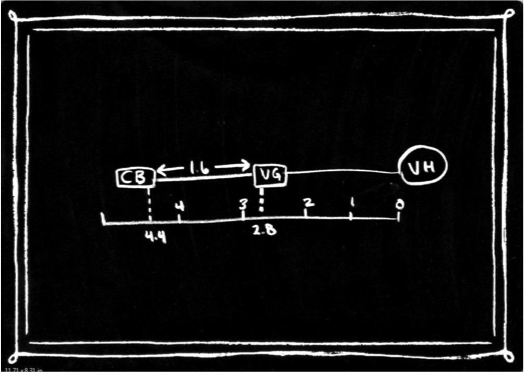## Diagrams

Although there are a number of problem-solving strategies that students use in mathematics, good problem solvers usually construct a representation of the problem to help them comprehend it (van Garderen & Montague, 2003). Students with LDs can have an especially challenging experience solving problems in math, and research suggests that they use visual representations less frequently (Montague, 1997) and less effectively (van Garderen et al., 2012) than their peers.

Generating visual representations, like diagrams, to solve math problems can help learners in numerous ways. Diagrams are effective for students with LDs as they can help highlight essential information and leave out information that is not necessary for solving a problem. This can simplify the problem-solving process (Kolloffel et al., 2009).

Perhaps the most consistent message in the literature about visual representation in mathematics is that it needs to be explicitly taught to students. Representing information visually is not a skill that comes naturally to students, and so it must be taught and practiced.

According to van Garderen and Scheuermann (2015), there are two distinct phases to teaching students how to use diagrams to solve word problems:

### Phase 1: Understanding Diagrams

Before learning how to use diagrams to solve problems, students need to learn how to create diagrams. Students may think that diagrams are simply visual representations of the problem and not understand that diagrams display the relational and quantitative information of the problem.

Ineffective diagrams, known in the literature as pictorial diagrams, depict the visual appearance of variables in the word problem (e.g., a drawing of a character in a word problem). Effective diagrams, known as schematic diagrams, go beyond visualizing the objects in the problem; they represent the content of the problem and depict the relational information (van Garderen, 2007). Schematic diagrams are extremely helpful for word problems in math and can be transferred across topics in mathematics, including geometry and probability, and across grades (Zahner & Corter, 2010).

### Phase 2: Using Diagrams

Once students understand that diagrams are cognitive tools that stand in the place of, and reflect, a thinking process, they need to learn how to use them to solve math problems.

During the second phase, students need to learn that using diagrams requires a three-step process:

1. Ask: What needs to be done?
2. Do: Produce a diagram.
3. Check: Confirm the diagram helps solve the problem.

While these steps may appear linear, students need to know that these steps are iterative (van Garderen & Scheuermann, 2015).

#### Example

The following example of a word problem illustrates the differences between pictorial and schematic diagrams and demonstrates how effective diagrams are tools for solving the problem.

The comic store is 4.4 kilometres west from Vera’s house. The video game store is 2.8 kilometres west from Vera’s house. How far is the comic store from the video game store?

Pictorial Diagram:Students with LDs may make the mistake of focusing too much attention on the details of the drawings. This student may have spent too much time drawing the buildings and including extraneous information, such as the compass. The unnecessary attention to detail may have been why the student mislabelled the distances.

Schematic Diagram:This student used a diagram that includes measures of length. By including relational information such as the distance on the number line, this student was better able to conceptualize and solve the problem.